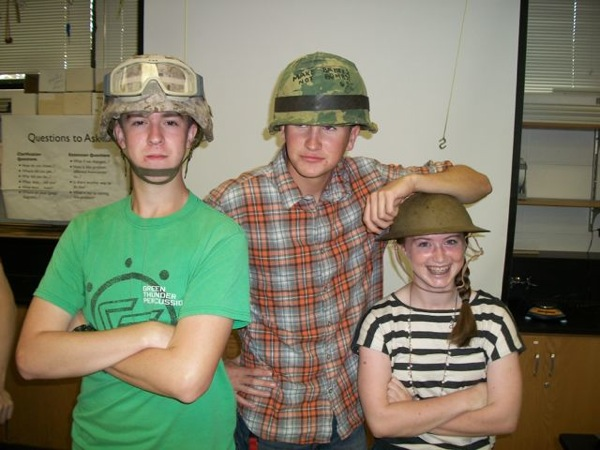# Projectile Motion Lab Practicum and Computational Modeling

In my AP Physics B class, I’m reviewing all of the material on the AP exam even though all of the students studied some of this materials last year in either Physics or Honors Physics. When we do have a review unit, I try to keep it engaging for all students by studying the concepts from a different perspective and performing more sophisticated labs.

When reviewing kinematics, I took the opportunity to introduce computational modeling using VPython and the physutils package. I started with John Burk’s Computational Modeling Introduction and extended it with my experiences at Fermilab where computational modeling plays a role in everything from the optics of interferometers to the distribution of dark matter in the galaxy. I then provided students with a working example of a typical projectile motion model and let them explore. I encouraged them to extend the model to have the projectile launched with an initial vertical displacement.

Later that unit, I introduced the lab practicum which was based on a lab shared by my counterpart at our neighboring high school. The goal of the lab was to characterize the projectile launcher such that when the launcher is placed on a lab table, the projectile will hit a constant velocity buggy driving on the floor, away from the launcher, at the specified location. The location would not be specified until the day of the lab practicum. No procedure was specified and students decided what they needed to measure and how they wanted to measure it. I also used this as practice for writing clear and concise lab procedures like those required on the free response section of the AP exam.

All groups figured out that they needed to determine the velocity of the car (which some had done the previous year) and the initial velocity of the projectile. Some groups used a technique very similar to the previous year’s projectile motion lab where a marble is rolled down a ramp and launched horizontally. These groups fired the projectile horizontally from atop the table and measured the horizontal displacement. Groups that calculated the flight time based on the vertical height were more accurate than those that timed the flight with a stopwatch. Another group fired the projectile straight up, measured the maximum height, and calculated the initial velocity. This group was particularly successful. Another group attempted to use a motion sensor to measure the initial velocity of the ball as they fired it straight up. The motion sensor had trouble picking up the projectile and this group’s data was suspect. A couple of other groups fired the projectile at a variety of angles, timed the flight, and measured the horizontal displacement. Some of these groups later realized that they didn’t really need to perform measurements at a variety of angles. After gathering data and calculating the initial velocity of the projectile as a group, I asked the students to practice calculating their launch angle based on a sample target distance. I hadn’t really thought this lab through and didn’t appreciate how challenging it would be to derive an equation for the launch angle as a function of horizontal displacement when the projectile is launched with an initial vertical displacement. It wasn’t until that night that I appreciated the magnitude of this challenge and then realized how this challenge could be used to dramatically improve the value of this lab.

Most students returned the next day a bit frustrated but with an appreciation of how hard it is to derive this equation. One student, who is concurrently taking AP Physics B and AP Physics C, used the function from his AP Physics C text successfully. Another student amazed me by completing pages of trig and algebra to derive the equation. No one tried to use the range equation in the text, which pleased me greatly (the found candy discussion must have made an impact on them). As we discussed how challenging it was to solve this problem, I dramatically lamented, “if only there was another approach that would allow us to solve this complex scenario…” The connection clicked and students realized that they could apply the computational model for projectile motion to this lab. Almost all of the groups chose to use the computational model. One student wrote his own model in Matlab since he was more familiar with that than Python. With assistance, all groups were able to modify the computational model and most were successful in hitting the CV buggy. One group dressed for the occasion:Students’ reflections on this lab were very positive. They remarked how they appreciated learning that there are some physics problems that are not easily solved algebraically (they are accustomed to only being given problems that they can solve). They also remarked that, while they didn’t appreciate the value of computational modeling at first, using their computational model in the lab practicum showed them its value. I saw evidence of their appreciation for computational modeling a couple of weeks later when a few of the students tried to model an after-school Physics Club challenge with VPython. For me, I was pleased that an oversight on my part resulted in a much more effective unit than what I had originally planned.